Question

# Write a program that asks the user to enter number, and displays all the numbers that...

Write a program that asks the user to enter number, and displays all the numbers that are multiples of 2 and 5 smaller than or equal to the number entered by the user.

Hint: A number n is a multiple of 2 if the remainder of the division of n by 2 is equal to zero.

Your program should have an output similar to the following:

Please enter a number: 50

The multiples of 2 and 5 less than or equal to 50 are: 10 20 30 40 50

in Java

Given below is the code in Java:

import java.util.Scanner; // import library for the Scanner classs

public class Main
{
public static void main(String[] args) {

Scanner sc = new Scanner(System.in); // define object of the scanner class
System.out.print("Please enter a number: ");
int number = sc.nextInt(); // take integer input from the user and assign it to number

System.out.print("The multiples of 2 and 5 less than or equal to 50 are: ");

for(int i=1;i<=number;i++) // Run loop from 1 till number. Value of i increments in every iteration.
{
if(i%2==0 && i%5==0) // if i is divisible by 2 and by 5 then print i. Mod (%) returns the remainder.
System.out.print(" "+i);
}

}
}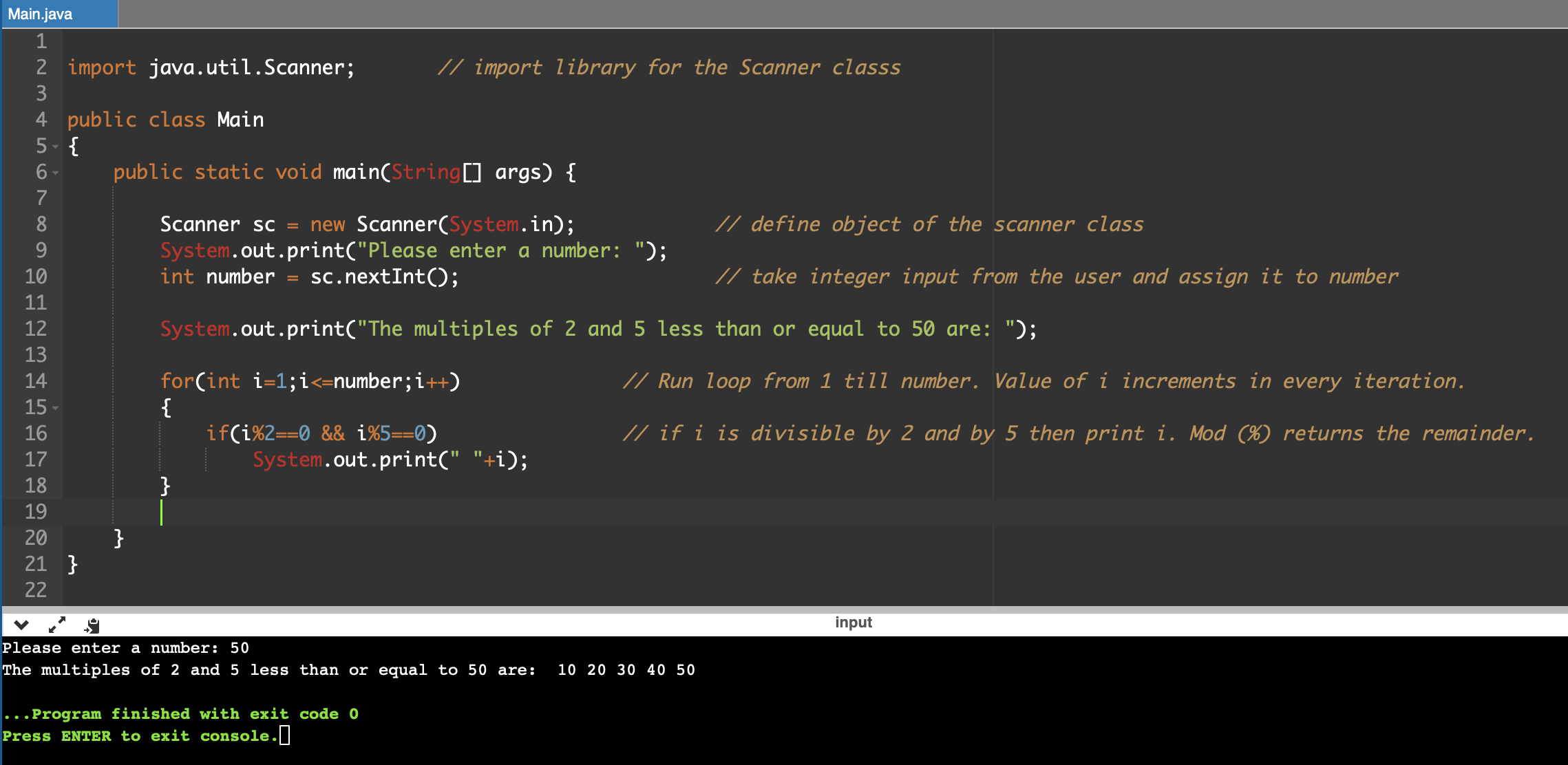#### Earn Coins

Coins can be redeemed for fabulous gifts.

Similar Homework Help Questions
• ### Write a program that asks the user to enter a number ofseconds.• There are...

Starting Out with C++ (9th Edition)  Chapter 4, Problem 7PCWrite a program that asks the user to enter a number of seconds.• There are 86400 seconds in a day. If the number of seconds entered by the user is greater than or equal to 86400, the program should display the number of days in that many seconds.• There are 3600 seconds in an hour. If the number of seconds entered by the user is less than 86400, but is greater...

• ### HELP IN JAVA: WHILE LOOP: Write a program that asks the user to enter a number...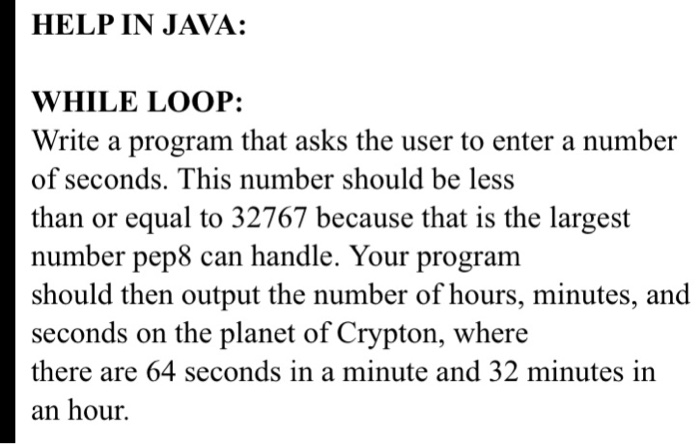HELP IN JAVA: WHILE LOOP: Write a program that asks the user to enter a number of seconds. This number should be less than or equal to 32767 because that is the largest number pep8 can handle. Your program should then output the number of hours, minutes, and seconds on the planet of Crypton, where there are 64 seconds in a minute and 32 minutes in an hour.

• ### Write a program that asks the user to enter two numbers. The program should then print on the...

Write a program that asks the user to enter two numbers. The program should then print on the screen one of the following messages depending on the values of thetwo numbers:a) the first number is smaller than the second numberb) the first number is larger than the second numberc) the first number is equal to the second numberI'm not exactly sure how to do the c++ while statements. Thank you very much for the help!

• ### Summary: Write a C program that prompts the user to enter 2 positive integer numbers, display...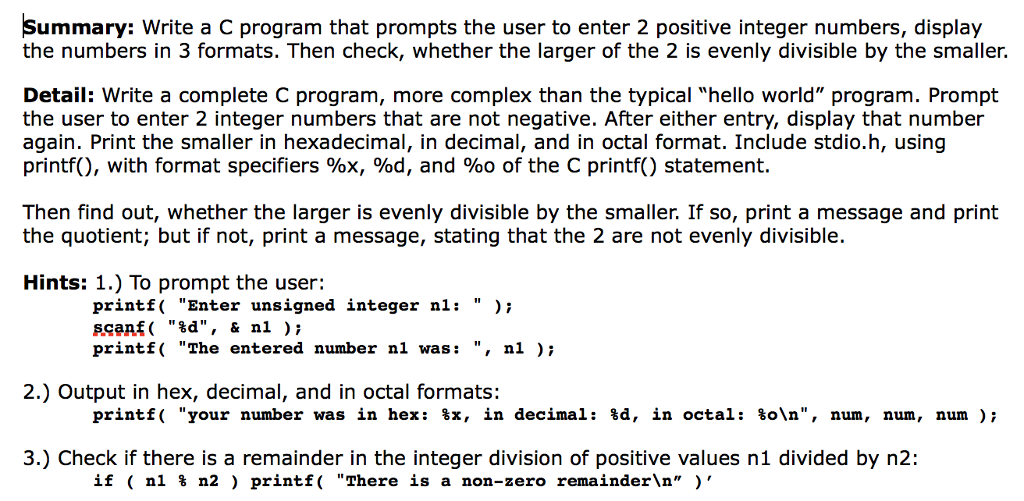Summary: Write a C program that prompts the user to enter 2 positive integer numbers, display the numbers in 3 formats. Then check, whether the larger of the 2 is evenly divisible by the smaller. Detail: Write a complete C program, more complex than the typical "hello world" program. Prompt the user to enter 2 integer numbers that are not negative. After either entry, display that number again. Print the smaller in hexadecimal, in decimal, and in octal format. Include...

• ### Write a java program that asks the user to enter an integer. The program should then...

Write a java program that asks the user to enter an integer. The program should then print all squares less than the number entered by the user. For example, if the user enters 120, the program should display 0, 1, 4, 9, 16, 25, 36, 49, 64, 81, and 100.

• ### Write an assembler program that asks the user (as shown below) for two integers and a...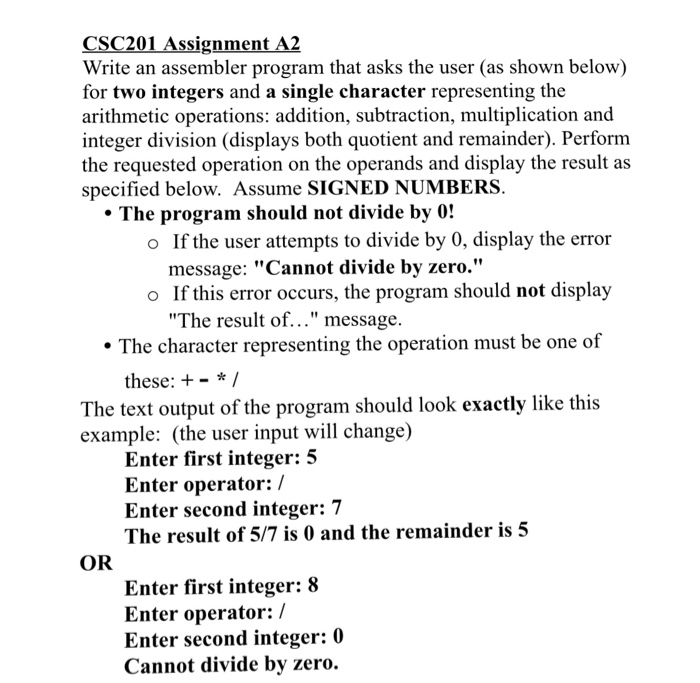Write an assembler program that asks the user (as shown below) for two integers and a single character representing the arithmetic operations: addition, subtraction, multiplication and integer division (displays both quotient and remainder). Perform the requested operation on the operands and display the result as specified below. Assume SIGNED NUMBERS. The program should not divide by 0! If the user attempts to divide by 0, display the error message: "Cannot divide by zero." If this error occurs, the program should...

• ### Write a C program that asks the user to enter two real numbers. Then your program...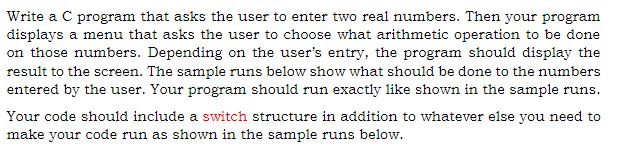Write a C program that asks the user to enter two real numbers. Then your program displays a menu that asks the user to choose what arithmetic operation to be done on those numbers. Depending on the user's entry, the program should display the result to the screen. The sample runs below show what should be done to the numbers entered by the user. Your program should run exactly like shown in the sample runs. make your code run as...

• ### java program: Write a program which displays the following list on the screen and asks the...

java program: Write a program which displays the following list on the screen and asks the user to enter either 1 or 2 and perform one of the operations based on the user’s input. If the user enters any other character other than 1 or 2 then display “wrong choice”. LIST OF OPERATIONS 1. Buzz Number                      2. Consecutive Odd numbers Note: A BUZZ number is a number which either ends with 7 or is divisible by 7. Sample input 27...

• ### Write a C program that asks the user to enter three numbers (integres). A menu will...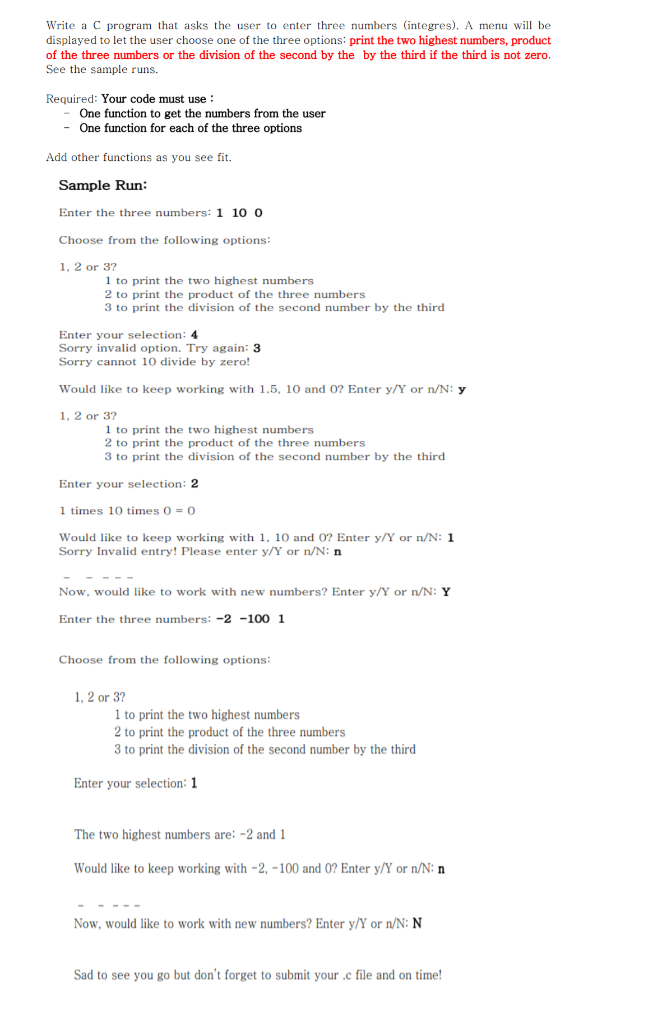Write a C program that asks the user to enter three numbers (integres). A menu will be displayed to let the user choose one of the three options: print the two highest numbers, product of the three numbers or the division of the second by the by the third if the third is not zero. See the sample runs. Required: Your code must use -One function to get the numbers from the user One function for each of the three...

• ### Write a java program that asks the user to enter 2 integers, obtains them from the...

Write a java program that asks the user to enter 2 integers, obtains them from the user, determines if they are even or odd numbers and prints their sum, product, difference, and quotient (Division).